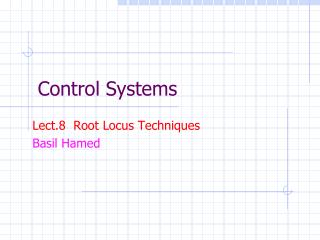# Control Systems - PowerPoint PPT PresentationDownload PresentationControl Systems

Control SystemsDownload Presentation## Control Systems

- - - - - - - - - - - - - - - - - - - - - - - - - - - E N D - - - - - - - - - - - - - - - - - - - - - - - - - - -
##### Presentation Transcript

1. Control Systems Lect.8 Root Locus Techniques Basil Hamed

2. Chapter Learning Outcomes After completing this chapter the student will be able to: Basil Hamed

3. Root Locus – What is it? • W. R. Evans developed in 1948. • Pole location characterizes the feedback system stability and transient properties. • Consider a feedback system that has one parameter (gain) K > 0 to be designed. • Root locus graphically shows how poles of CL system varies as K varies from 0 to infinity. L(s): open-loop TF Basil Hamed

4. Root Locus – A Simple Example Characteristic eq. K = 0: s = 0,-2 K = 1: s = -1, -1 K > 1: complex numbers Basil Hamed

5. Root Locus – A Complicated Example Characteristic eq. • It is hard to solve this analytically for each K. • Is there some way to sketch a rough root locus by hand? Basil Hamed

6. 8. 1 Introduction • Root locus, a graphical presentation of the closed-loop poles as a system parameter is varied, is a powerful method of analysis and design for stability and transient response(Evans, 1948; 1950). • Feedback control systems are difficult to comprehend from a qualitative point of view, and hence they rely heavily upon mathematics. • The root locus covered in this chapter is a graphical technique that gives us the qualitative description of a control system's performance that we are looking for and also serves as a powerful quantitative tool that yields more information than the methods already discussed. Basil Hamed

7. 8.2 Defining the Root Locus The root locus technique can be used to analyze and design the effect of loop gain upon the system's transient response and stability. Assume the block diagram representation of a tracking system as shown, where the closed-loop poles of the system change location as the gain, K, is varied. Basil Hamed

8. 8.2 Defining the Root Locus The T.F shows the variation of pole location for different values of gain k. Pole location as a function of gain for the system Basil Hamed

9. 8.4 Sketching the Root Locus Obtain the open-loop function kG(s)H(s) Characteristic Eq.: 1+kG(s)H(s)=0 Mark Poles with X and Zeros with O Draw the locus on the real axis to the left of an odd number of real poles plus zeros. The R-L is Symmetrical with respect to the real axis. Basil Hamed

10. 8.4 Sketching the Root Locus • The R-L originates on the poles of G(s)H(s) and terminates on the zeros of G(s)H(s) • Draw the asymptotes α = n – m α :numb of asymptotes, n: numb of zeros, m: numb of poles 1+kG(s)H(s) = 0, k= • The break away points will appear among the roots of polynomial obtained from: = 0 OR -D(s) Basil Hamed

11. Example Find R-L Basil Hamed

12. Example Sketch R-L Solution: Indicate the direction with an arrowhead Basil Hamed

13. Example Intersections of asymptotes = Asymptotes (Not root locus) Breakaway points are among roots of s = -2.4656, -0.7672 ± 0.7925 j Basil Hamed

14. Example Breakaway point -2.46 K=.4816 Basil Hamed

15. Root Locus – Matlab Command “rlocus.m” Basil Hamed

16. Example There are three finite poles, at s = 0, — 1, and - 2, and no finite zeros Basil Hamed

17. Example Basil Hamed

18. Example 8.2 P. 400 PROBLEM: Sketch the root locus for the system shown in Figure • SOLUTION: Let us begin by calculating the asymptotes α = n – m =4-1=3 • =±60,+ 180 • Breakaway point= -D(s)==-.44 Basil Hamed

19. Example 8.2 P. 400 Basil Hamed

20. Root-locus diagrams that show the effects of adding poles to G(s) H(s) a>0 • b>a>0 Basil Hamed

21. Root-locus diagrams that show the effects of adding poles to G(s) H(s) another pole is added to G(s)H(s) at s = -c • addition of a pair of complex conjugate • poles to the transfer function Basil Hamed

22. Root-locus diagrams that show the effects of adding a zero to G(s)H(s) Basil Hamed

23. Example Given :find R-L when b=1,i) a=10, ii)a=9, iii)a=8 , iv) a=3, v) a=1 Solution: i)a = 10. Breakaway points: s = -2.5 and -4.0. Basil Hamed

24. Example ii) a = 9. The breakaway point at s = -3. iii) a = 8. No breakaway point on RL Basil Hamed

25. Example iv) a = 3. v) a = b = 1. The pole at s = -a and the zero at -b cancel each other out, and the RL degenerate into a second-order case and lie entirely on the jw-axis. Basil Hamed

26. Example Consider the closed loop system with open loo function K a) sketch R-L b)What range of k that ensures stability? Solution: Basil Hamed

27. Example Not valid Basil Hamed

28. Example Part b) CharctEq, 1+kGH=0 1+=0 Using R-H array For stability need b= (-1/4)(k-10)>0 k<10 C= k-6 k> 6 6<k<10 Basil Hamed

29. Example Find R-L and find k for critical stability Solution Breakaway points are among roots of Basil Hamed

30. Example Basil Hamed

31. Example Characteristic equation Routh array When K = 30 Basil Hamed

32. Example Basil Hamed